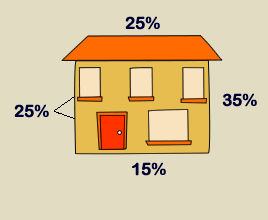Buying a Home Home Improvements Home Living Home Costs Selling your home

# Heatloss CalculationThe heat loss calculation indicated below is a simple example of how the heat requirement is calculated for a room.

First the air volume must be heated – Measure the room length height and width in metres   and multiply by 0.33 and then by the number of air changes an hour you expect that room to have. Generally this will vary from 1 to 2 depending on its location and use.

The next step is to calculate the heat loss through the windows. Measure the area of the windows in square metres and then multiply by the thermal properties of the window ie. The ‘U’ value for a single  glazed window the ‘U’ value will be 5.8 w/m2/degC and 2.9 for double glazed windows. If you have very special glazing system the ‘U’ value may be different so check with the manufactures.

The heat loss through the external walls is calculated similar to the windows. Measure the area of the external wall and multiply by the ‘U’ value which could range from 1.5 for a conventional cavity brick wall to 0.3 if highly insulated. Again you will need to check with the manufactures.

Heat losses through the ground floor and roof are also calculated in a similar way. When all the surfaces which lose heat have been calculated, add up the total and also include the air volume. This will give you a value fore the room expressed in watts/degC.  Multiply this by the maximum temperature you wish to heat the room usually 21 degrees C. with an outside temperature of –1degreeC. This represents a temperature rise of 22 deg C. This value will now be the heat required in Watts to heat the room. If you want this value in kW the just divide by 1000.

Example calculation for heat loss

(Ground floor room with a heated room above ie. no heat loss through the ceiling)

 Example Heat Loss calulation

Length

Width

Height

Factor

Air Changes

w/degC

Air volume

3.3

3

2.3

0.33

2

15.03

Glass

3

2

2.9

17.4

Ext Wall

6

2.3

1.5

20.7

Floor

3.3

3

1

9.9

Total

63.03

Room temperature required  21 degree C when -1 outside=

1386.62

Watts